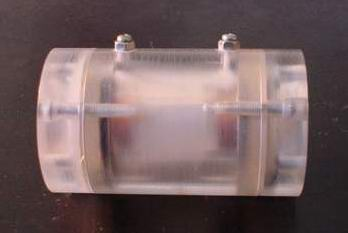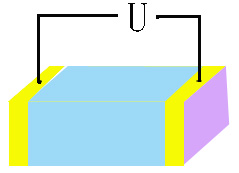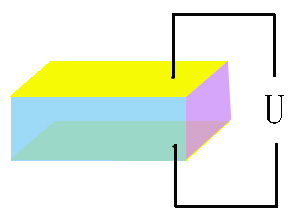﻿ E-O crystalsCrystalsQ-SwitchE-O Crystals

Introduction

Electro-optic Pockels cells are used in applications that require fast switching of the polarization direction of a beam of light. These uses include Q-switching of laser cavities, coupling light into and out from regenerative amplifiers, and, when used in conjunction with a pair of polarizers, light intensity modulation. Pockels cells are characterized by fast response, since the Pockels Effect is largely an electronic effect that produces a linear change in refractive index when an electric field is applied, and are much faster in response than devices based on acoustic changes in a material, for example.

When an electric field (E) is applied to an electro-optic (E-O) crystal, the refractive index of E-O crystal willchange linearly to electric field. The phenomenon is called linear electro-optic effect. For KD*P crystal, for example, the change of the refractive index (Dn) is Dn = 0.5n3or63E if both the directions of light propagation and electric field are along the z-axis, where no is refractive index without electric field and r63 is electro-optic coefficient of KD*P.

If a linearly polarized light passes through an E-O crystal, the phase retardation (G) will be induced by Dn to G = 2pDnL, where L is crystal length, for KD*P, again as an example, G = pLn3or63E/l. It is clear that the phase of light will change together with electric field (E). This is called electro-optic phase modulation. If two crossed polarizers are placed at input and output ends of E-O crystal separately, the output intensity of light will be I = I0sin2(G/2), where I0 is input intensity. That means the intensity or amplitude of light can also be modulated by electric field. This is called amplitude modulation.longitudinal E-O modulation transverse E-O modulation

There are two kinds of E-O modulations. One is longitudinal E-O modulation if the directions of electric field and light propagation are the same. The KDP isomorphic crystals are normally used in this scheme. If the directions of electric field and light propagation are perpendicular, it is called transverse E-O modulation. The LiNbO3, MgO:LiNbO3, ZnO:LiNbO3, BBO and KTP crystals are usually employed in this scheme.

The half-wave voltage (Vp) is defined as the voltage at G = p, for example, Vp=l/(2no3r63) for KD*P and Vp=ld/(2no3r22L) for LiNbO3, where l is light wavelength and d is the distance between the electrodes.

Electro-Optic Crystals and Acousto-Optic Crystals: LiTaO3; BSO; BGO; TeO2; PbMoO4, etc.